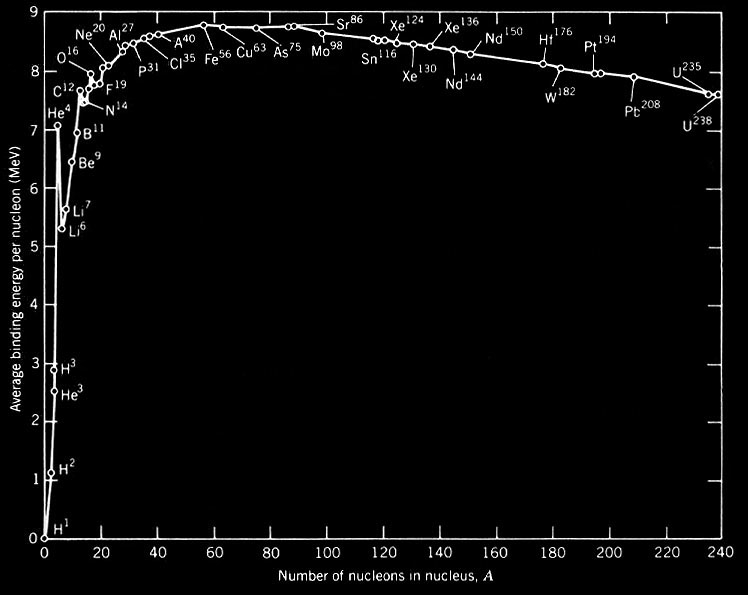As mentioned when we looked at energy generation, it is now known that most of the energy radiated by stars must be released by nuclear reactions. In this section we will consider why it is that energy can be released by nuclear reactions.

Atomic nuclei are composed of protons and neutrons (or nucleons). The total mass of a nucleus is less than the mass of its constituent nucleons. This means that if a compound nucleus is formed from nucleons there is a loss of mass which, by Einstein's mass-energy relation, E=mc2, is released as energy. This energy is known as the binding energy. The binding energy, Q(Z,N), of a nucleus composed of Z protons and N neutrons is:

Q(Z,N) = [Zmp+Nmn- m(Z,N)]c2,

where mp is the proton mass, mn the neutron mass and m(Z,N) the mass of the compound nucleus. The more stable the nucleus, the greater the energy that must be supplied to unbind it, or equivalently, the greater the energy that is released when it is formed. A more useful measure of stability is the binding energy per nucleon, Q(Z,N)/(Z+N). This is the energy needed to remove an average nucleon from the nucleus and is proportional to the fractional loss of mass when the compound nucleus is formed. Figure 14 shows a plot of the average binding energy per nucleon versus the atomic mass number, A = Z+N. It can be seen that the curve increases rapidly with low A, hits a broad maximum for atomic mass numbers of 50 to 60 (corresponding to nuclei in the neighbourhood of iron in the periodic table, which are the most strongly bound nuclei) and then gradually declines for nuclei with higher values of A.

 Figure 14: Binding energy per nucleon plotted as a function of the atomic mass number.Figure 14 shows how nuclear fusion and fission reactions can release energy. If two nuclei lying to the left of the maximum in figure 14 fuse to form a compound which also lies to the left of the maximum, the compound nucleus will have a larger binding energy per nucleon than the original nuclei. As the total number of nucleons has not been changed, such nuclear fusion reactions must release energy. Once the compound nucleus lies in the iron region of figure 14, however, further energy release from fusion reactions becomes impossible. Thus if a star initially consisted of pure hydrogen, it could generate a maximum of about 9 MeV per nucleon by fusion to 56Fe, but about 7 MeV of this would have already been released when 4He is formed during the first step. (Note that 1 eV = 1.6x10-19 J.)

If a heavy nucleus lying to the right of the maximum in figure 14 splits into two or more fragments which also lie to the right of the maximum, the newly formed nuclei will have a larger binding energy per nucleon than the original nucleus. Such nuclear fission reactions must therefore also release energy. However, the binding energy per nucleon of very heavy nuclei, although less than that of iron, is still quite large. This means that the maximum possible energy release per kg from fission reactions is much less than that from fusion reactions. Coupled with the fact that very heavy nuclei do not appear to be very abundant in nature, we may conclude that nuclear fusion reactions are by far the most important source of energy generation in stars.